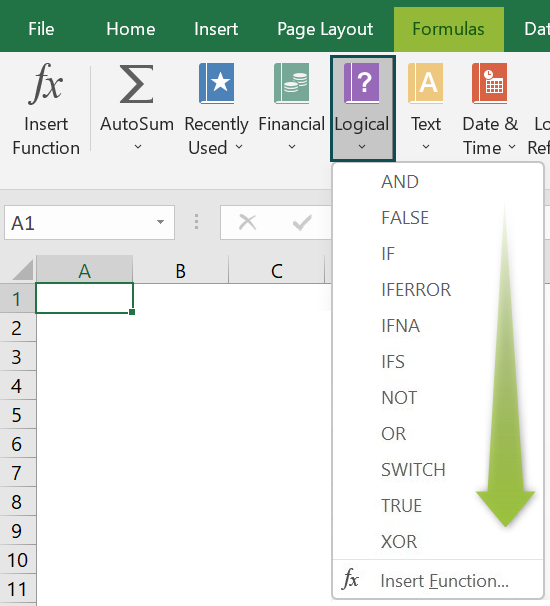# Logical Test In ExcelArticle byExcelMojo Team## What Is Logical Test In Excel?

The Logical Test in Excel checks for the given criteria/condition and displays the required result, either TRUE, FALSE, or any other conditioned result. We can perform Logical Tests using the Excel function such as IF, AND, OR, NOT, XOR, etc.

The functions w.r.t the Excel Logical Test are categorized under the Logical functions of the Function Library.

###### Key Takeaways
• Logical tests in Excel are used to find the logical, TRUE, or FALSE, results for the calculations.
• The formulas include conditions using Logical Operators like >, <, =, <>, etc., or they can be the results of arithmetic calculations.
• Different logical tests follow different logic in Excel.
• If the function returns one value if the condition is satisfied and another if not. The Logical Tests result will always be the default TRUE or FALSE.
• The output can be altered to give a conditioned output, i.e., we can insert an output in the formula to give an alternate value as we considered in Example 2 where instead of a “#NAME?” error, we get the output as “Wrong Formula Entered”.

### Top 10 Types Of Logical Tests In Excel

The types of Logical Tests in Excel are as follows:

• The AND excel function gives the output as TRUE when all the selected argument cell values estimate TRUE, and returns FALSE if one or more arguments estimate FALSE.

The syntax of the AND function is =AND(logical1,logical2).

• The FALSE excel function is the Boolean Logical Function equal to 1. The function is equivalent to directly using the Boolean value FALSE in a formula.

Here, the syntax of the False function is =FALSE().

• The IF excel function tests whether the condition is fulfilled and returns the result as “true” and “false” for the logical text or value.

The syntax of the IF function is =IF(logical_test,value_if_true,value_if_false).

• The IFERROR excel function handles many excel errors, like #N/A, #NUM!, #NAME?, etc., and returns the value TRUE if any of these errors are found.

Here, the syntax of the IFERROR Function is; =IFERROR(value,value_if_error).

• The IFNA function checks if any of the cell value or the result of a formula is a #N/A error and returned the results accordingly.

The syntax of the IFNA function is =IFNA(value,value_if_na).

• The IFS excel function detects whether one or more conditions are fulfilled and returns a value corresponding to the first TRUE condition.

Here, the syntax of the IFS function is =IFS(logical_test,value_if_true).

The syntax of the NOT function is =NOT(logical).

• The OR excel function returns TRUE if any of the one argument is TRUE and returns FALSE if all the arguments are FALSE.

Hers, the syntax of the OR function is =OR(logical1,logical2).

• The SWITCH excel function checks a cell reference or cell value against all the values in the selected dataset, and returns the result as per the first match.

The syntax of the SWITCH function is =SWITCH(expression,value1,result1,default_or_value2,result2).

• The TRUE excel function is the Boolean Logical Function equal to 1. The function is equivalent to directly using the Boolean value TRUE in a formula.

Here, the syntax of the TRUE function is =TRUE().

The syntax of the XOR function is =XOR(logical1,logical2).

### How To Use Logical Tests In Excel?

We can use the Logical Tests In Excel in 2 ways, namely:

1. Enter the Logical functions in the worksheet manually.
2. Access the Logical functions from the Excel ribbon.

#### Method #1 – Enter the Logical functions in the worksheet manually

1. Select an empty cell for the output.
2. Type “=” followed by the Logical function name in the selected cell. [Alternatively, type the starting letter of the required Logical function and double-click the function from the list of suggestions shown by Excel.]
3. Enter the arguments as cell values or cell references.
4. Press the “Enter” key.

#### Method #2 – Access the Logical functions from the Excel ribbon

Choose an empty cell for the result → select the “Formulas” tab → go to the “Function Library” group → click the “Logical” option drop-down → select the required function, as shown in the below image and explained in the previous section.

For example, we will calculate the logical condition of the given data using the Logical Tests IF Excel.

In the table, the data is,

• Column A: contains Name.
• Column B: contains Output.

The steps to calculate the combination using the IF function are as follows:

1. Select cell B2, enter the formula =IF(A2=“John”,“TRUE”), and press the “Enter” key. The result is “TRUE”, as shown below.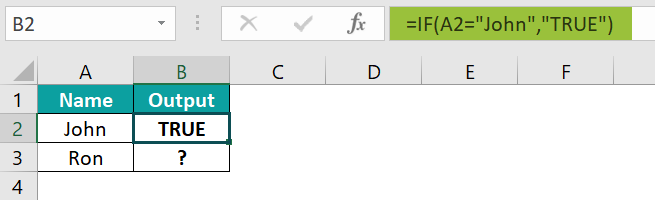2. Drag the formula from cell B2 to B3 using the fill handle. The output is shown below.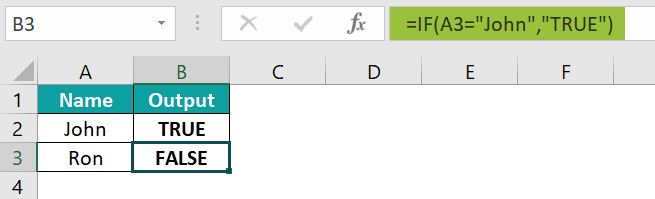### Examples

We will consider some scenarios to use the Logical Tests in Excel.

#### Example #1 – AND Logical Test Function

For example, we will find the Logical Test in Excel using the AND function for the given data.

In the table, the data is,

• Column A: contains Value.
• Column B: contains Output.

The procedure to calculate the combination using the AND function is,

Select cell B2, enter the formula =AND(A2=10,A2=20), and press the “Enter” key.

The output is shown above.

#### Example #2 – IFERROR Logical Test Function

For example, there is a list of numbers, and the sum of those numbers is calculated.

In the table, the data is,

• Column A: contains Numeric value.
• Column B: contains Output.

The steps to calculate the combination using the IFERROR function are as follows:

• Step 1: Select cell C2,and enter the formula =su(A2:A10).

The Wrong function name of the sum is entered. The entered formula is “su(A2:A10)”, but it should be “SUM(A2:A10)” due to this “#NAME?” error is generated.

• Step 2: We will use the IFERROR function, and replace the “#NAME?” with the “Wrong Formula Entered” text string.

Now, enter the new formula =IFERROR(su(A2:A10),”Wrong Formula Entered”), as shown below.

We get the above output as “Wrong Formula Entered” in place of the error.

#### Example #3 – TRUE Logical Test Function

For example, we will calculate the logical condition of the data using the TRUE Logical function.

In the table, the data is,

• Column A: contains Output.
• Column B: contains Formula.

The steps to calculate the combination using the TRUE function are as follows:

• Step 1: Select cell A2, enter the formula =TRUE, and press the “Enter” key. The result is “TRUE”.
• Step 2: Drag the formula from cell A2 to A3 using the fill handle.

The output is shown above. Column B is for our reference.

### Important Things To Note

• The AND function requires all the logical test conditions to be TRUE. If any condition is not satisfied, it will return FALSE.
• The OR function requires at least any logical test condition to be TRUE.
• The IFERROR function, NOT function, TRUE Function, FALSE function, etc… are also part of the Logical functions.
• The output of the XOR Excel logical test is TRUE, if only one condition fulfilled, and FALSE if both are fulfilled or not.
• The output of the NOT function is TRUE if a condition is not fulfilled, and FALSE the same condition is fulfilled.

1. What does the Logical Test do in Excel?

The Logical Test in Excel checks if one or more conditions are TRUE or FALSE.

2. How to Use FALSE Logical Tests in Excel?

For example, we will calculate the logical condition of the given data using the FALSE Logical Tests.

In the table, the data is,
• Column A: contains Value.
• Column B: contains Output.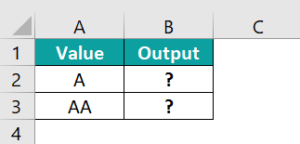The steps to calculate the combination using the FALSE function are as follows:

Step 1: Select cell B2, enter the formula =A2=“AA”, and press the “Enter” key. The result is “FALSE”.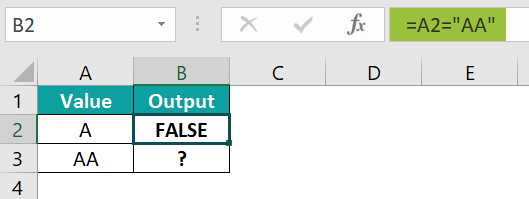Step 2: Drag the formula from cell B2 to B3 using the fill handle. The output is shown below.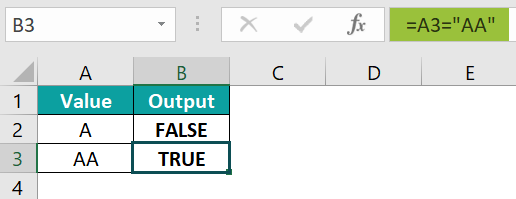3. Where is the Logical Test in Excel?

One can insert the function of the Logical Test in Excel as follows:

Choose an empty cell for the result → select the “Formulas” tab → go to the “Function Library” group → click the “Logical” option drop-down → select the required function, as shown below.